##### The Japanese Bonsai specialist
Direct order Contact Help / Services Newsletter# Stone lantern kotoji 140 cm

Lanterne kotoji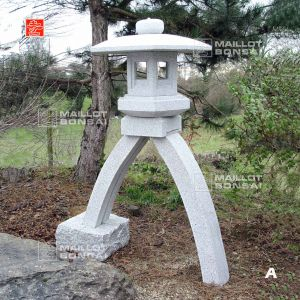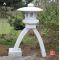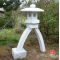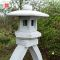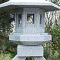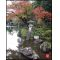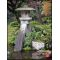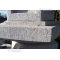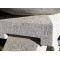ref. : 7431

710,00

voluminous and/or heavy product extra shipping of 75,00

This item is temporarily unavailableNotify availability

###### Description

Height 140 cm. Width of the top section 80 cm, weight approximately 365 kilograms delivered in 8 pieces to be assembled at home. This granite lantern style called kotoji is a copy of one that has become a symbol of the Kenrokuengarden in Japan. The shape of the legs is like a Japanese koto (harp) and one leg has a base that acts as a counterweight to balance the lantern. In Kenrokuen garden this impressive lantern is placed next to the shore of a pond in the water and floodlit at night – an idea you might like to emulate in your garden. A lamp can also be fitted to this lantern.

#lantern 8.7 #stone 4.5 #kotoji 3.6 #this 3.4 #garden 2.6 #kenrokuengarden 2.5 #like 2.4 #that 2.4 #approximately 2.3 #counterweight 2.3

Formule
(( ROUND((CHAR_LENGTH(b.article_nom)-CHAR_LENGTH(REPLACE(b.article_nom, 'lantern', '')))/LENGTH('lantern')) + ROUND((CHAR_LENGTH(b.article_description)-CHAR_LENGTH(REPLACE(b.article_description, 'lantern', '')))/LENGTH('lantern')) ) * 6.7) + (( ROUND((CHAR_LENGTH(b.article_nom)-CHAR_LENGTH(REPLACE(b.article_nom, 'kotoji', '')))/LENGTH('kotoji')) + ROUND((CHAR_LENGTH(b.article_description)-CHAR_LENGTH(REPLACE(b.article_description, 'kotoji', '')))/LENGTH('kotoji')) ) * 3.6) + (( ROUND((CHAR_LENGTH(b.article_nom)-CHAR_LENGTH(REPLACE(b.article_nom, 'this', '')))/LENGTH('this')) + ROUND((CHAR_LENGTH(b.article_description)-CHAR_LENGTH(REPLACE(b.article_description, 'this', '')))/LENGTH('this')) ) * 3.4) + (( ROUND((CHAR_LENGTH(b.article_nom)-CHAR_LENGTH(REPLACE(b.article_nom, 'garden', '')))/LENGTH('garden')) + ROUND((CHAR_LENGTH(b.article_description)-CHAR_LENGTH(REPLACE(b.article_description, 'garden', '')))/LENGTH('garden')) ) * 2.6) + (( ROUND((CHAR_LENGTH(b.article_nom)-CHAR_LENGTH(REPLACE(b.article_nom, 'stone', '')))/LENGTH('stone')) + ROUND((CHAR_LENGTH(b.article_description)-CHAR_LENGTH(REPLACE(b.article_description, 'stone', '')))/LENGTH('stone')) ) * 2.5) + (( ROUND((CHAR_LENGTH(b.article_nom)-CHAR_LENGTH(REPLACE(b.article_nom, 'kenrokuengarden', '')))/LENGTH('kenrokuengarden')) + ROUND((CHAR_LENGTH(b.article_description)-CHAR_LENGTH(REPLACE(b.article_description, 'kenrokuengarden', '')))/LENGTH('kenrokuengarden')) ) * 2.5) + (( ROUND((CHAR_LENGTH(b.article_nom)-CHAR_LENGTH(REPLACE(b.article_nom, 'like', '')))/LENGTH('like')) + ROUND((CHAR_LENGTH(b.article_description)-CHAR_LENGTH(REPLACE(b.article_description, 'like', '')))/LENGTH('like')) ) * 2.4) + (( ROUND((CHAR_LENGTH(b.article_nom)-CHAR_LENGTH(REPLACE(b.article_nom, 'that', '')))/LENGTH('that')) + ROUND((CHAR_LENGTH(b.article_description)-CHAR_LENGTH(REPLACE(b.article_description, 'that', '')))/LENGTH('that')) ) * 2.4) + (( ROUND((CHAR_LENGTH(b.article_nom)-CHAR_LENGTH(REPLACE(b.article_nom, 'approximately', '')))/LENGTH('approximately')) + ROUND((CHAR_LENGTH(b.article_description)-CHAR_LENGTH(REPLACE(b.article_description, 'approximately', '')))/LENGTH('approximately')) ) * 2.3) + (( ROUND((CHAR_LENGTH(b.article_nom)-CHAR_LENGTH(REPLACE(b.article_nom, 'counterweight', '')))/LENGTH('counterweight')) + ROUND((CHAR_LENGTH(b.article_description)-CHAR_LENGTH(REPLACE(b.article_description, 'counterweight', '')))/LENGTH('counterweight')) ) * 2.3)

## Secure payment## Delivery

Our logistic partners :